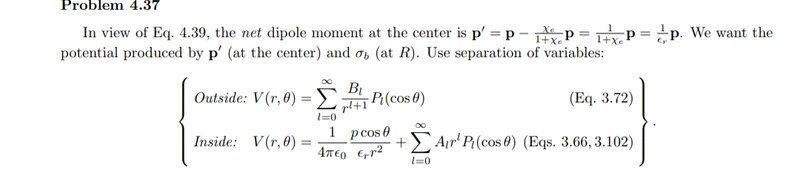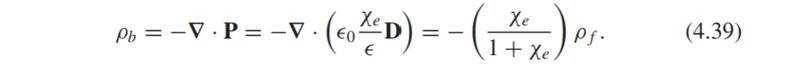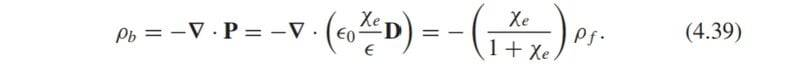# Griffith's problem 4.37 -- A point dipole at the center of a sphere of linear dielectric material

• Ahmed1029
In summary, the conversation discusses the solution for a problem involving dipole moments and Laplace's equation. The solution manual uses a complex ansatz that takes into account the multipole expansion and boundary conditions, but the speaker believes that a simpler solution with only dipole fields would suffice. However, the solution is still valid due to the use of superposition.

#### Ahmed1029

Homework Statement
A point dipole p is imbedded at the center of a sphere of linear
dielectric material (with radius R and dielectric constant er). Find the electric potential inside and outside the sphere.
Relevant Equations
Laplace's equation
I tried to solve it for some time and then looked at the solution manual, which got me completely lost. Those are the first lines of the solution :I'm not so sure how equation 4.39:makes him conclude that the same relation holds for dipole moments. My second concern is that I'm not sure how he uses laplace's eauation when the center of the sphere has a dipole consisting of equal and opposite charges. How am I allowed to solve laplace's equation in places where there is charge? Also I'm not sure how he got the potential inside the sphere. Did he use the superposition of the dipole potential with that of the surface charge bound potential? Someone help me please

•Delta2
The only source is the point dipole (or rather the "net dipole"). That's explicitly taken into account by the first term in the "Inside: equation". Then he superimposed a general solution of the Laplace equation in terms of the multipole expansion, where he already worked in the rotation symmetry around the ##x_3## axis, i.e., of all the spherical harmonics on the ##\text{Y}_{\ell0}## occur, and these he wrote in terms of the Legendre Polynomials instead.

Now, what's left to do is to fulfill the boundary conditions. He worked also in the obvious ones: inside the sphere the only singularity can be due to the "net dipole" in the origin, and this term he has written explicitly. The rest is the potential of the "induced" fields, which is from the continuous induced-dipole density in the medium and thus has no singularities at ##r=0##. That's why he only used the "increasing multipoles", i.e., for ##r^{\ell}## in front of the ##\ell##th harmonic. For ##r \rightarrow \infty## you can assume that the potential goes to 0 since there are only sources within a the sphere, i.e., no charge distributions extending to infinity. That's why it's sufficient to assume only the "decreasing multipoles", i.e., ##1/r^{\ell+1}## in front of the ##P_{\text{ell}}##.

All that's left to do is to fulfill the boundary conditions at the surface of the dielectric. Since there's no free charge on the surface thus ##\vec{n} \cdot \vec{D}=\vec{r}/r \cdot \vec{D}## (normal component of ##\vec{D}## along the surface) must be continuous (a jump would be the free surface charge, but there's none) and of course the potential itself must be continuous.

I think, however the ansatz in the solution is a bit too complicated, because due to symmetry it's pretty clear that you only need dipole fields everywhere, because there's no other source there than a dipole and the sphere is, well, spherically symmetric. Of course this also comes out immediately from the general ansatz.

••Delta2 and Ahmed1029
vanhees71 said:
The only source is the point dipole (or rather the "net dipole"). That's explicitly taken into account by the first term in the "Inside: equation". Then he superimposed a general solution of the Laplace equation in terms of the multipole expansion, where he already worked in the rotation symmetry around the ##x_3## axis, i.e., of all the spherical harmonics on the ##\text{Y}_{\ell0}## occur, and these he wrote in terms of the Legendre Polynomials instead.

Now, what's left to do is to fulfill the boundary conditions. He worked also in the obvious ones: inside the sphere the only singularity can be due to the "net dipole" in the origin, and this term he has written explicitly. The rest is the potential of the "induced" fields, which is from the continuous induced-dipole density in the medium and thus has no singularities at ##r=0##. That's why he only used the "increasing multipoles", i.e., for ##r^{\ell}## in front of the ##\ell##th harmonic. For ##r \rightarrow \infty## you can assume that the potential goes to 0 since there are only sources within a the sphere, i.e., no charge distributions extending to infinity. That's why it's sufficient to assume only the "decreasing multipoles", i.e., ##1/r^{\ell+1}## in front of the ##P_{\text{ell}}##.

All that's left to do is to fulfill the boundary conditions at the surface of the dielectric. Since there's no free charge on the surface thus ##\vec{n} \cdot \vec{D}=\vec{r}/r \cdot \vec{D}## (normal component of ##\vec{D}## along the surface) must be continuous (a jump would be the free surface charge, but there's none) and of course the potential itself must be continuous.

I think, however the ansatz in the solution is a bit too complicated, because due to symmetry it's pretty clear that you only need dipole fields everywhere, because there's no other source there than a dipole and the sphere is, well, spherically symmetric. Of course this also comes out immediately from the general ansatz.

So my conclusion is that the potential for the "inside" of the sphere, written this way, won't satisfy laplace's equation everywhere within the sphere, but the solution is fine only beceuase he used the superposition of the potentials, which will in turn satisfy Poisson's equation in the place of the dipole, is that right? Or Did he just consider the dipole to he one point of zero charge and then laplace's equation is fine?

Ahmed1029 said:
Homework Statement:: A point dipole p is imbedded at the center of a sphere of linear
dielectric material (with radius R and dielectric constant er). Find the electric potential inside and outside the sphere.
Relevant Equations:: Laplace's equation

I'm not so sure how equation 4.39:makes him conclude that the same relation holds for dipole moments.
The ##\rho_f## of the dipole which has a dipole moment ##\mathbf{p}=\int \rho_f (\mathbf{r}-\mathbf{r'})d^3\mathbf{r'}## also creates a ##\rho_b## inside the dielectric according to 4.39 and this ##\rho_b## has a dipole moment ##\mathbf{q}=\int \rho_b (\mathbf{r}-\mathbf{r'}) d^3 \mathbf{r'}=-\frac{\chi_e}{1+\chi_e}\mathbf{p}##. The net dipole moment ##\mathbf{p'}=\mathbf{p}+\mathbf{q}=\frac{1}{1+\chi_e}\mathbf{p} ##.

•Ahmed1029 and vanhees71
Ahmed1029 said:
So my conclusion is that the potential for the "inside" of the sphere, written this way, won't satisfy laplace's equation everywhere within the sphere, but the solution is fine only beceuase he used the superposition of the potentials, which will in turn satisfy Poisson's equation in the place of the dipole, is that right? Or Did he just consider the dipole to he one point of zero charge and then laplace's equation is fine?
Of course at the place of the dipole you have a dipole source. We solve of course the Poisson equation with this singular source. The singular contribution of this dipole is considered explicitly, as described above.

•Ahmed1029